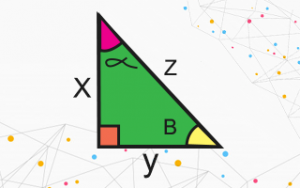# Class 10th - Introduction to Trigonometry (Hindi)

Simply Easy Learning

Published on 08/2019

0
• Introduction to Trigonometry
04:46
Preview
• Trigonometric Ratios
10:27
• Trigonometric Ratios of Complementary Angles
06:34
• Trigonometric Identities
08:22
• Introduction to Trigonometry Example 1
04:15
• Introduction to Trigonometry Example 2
06:45
• Introduction to Trigonometry Example 3
03:25
Preview
• Introduction to Trigonometry Example 4
08:56
• Introduction to Trigonometry Example 5
05:41

#### Description

Trigonometry is the study of relationships between the sides and angles of a triangle.

In this chapter, we will study ratios of the sides of a right triangle with respect to its acute angles, called trigonometric ratios and then we will calculate <b>trigonometric ratios</b> for some specific angles and establish some identities involving these ratios, called <b>trigonometric identities</b>.

# Audience

These video lectures have been prepared in Hindi exclusively for all English and Hindi medium state board and central board class 10th students to have the understanding of Trigonometry.

0
Course Rating
0%
0%
0%
0%
0%

#### Feedbacks (0)

• No Feedbacks Yet..!This Course Includes :

59 mins

9 Lectures